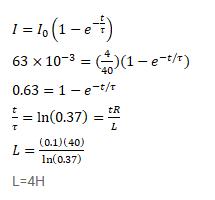# A coil of resistance 40Ω is connected across a 4.0 V battery 0.10s

Question:

A coil of resistance $40 \Omega$ is connected across a $4.0 \mathrm{~V}$ battery $0.10$ s after the battery is connected, the current in the coil is $63 \mathrm{~mA}$. Find the inductance of the coil.

Solution: